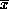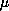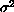Electronic Journal of Differential Equations 15th annual Conference of Applied Mathematics, Univ. of Central Oklahoma,
Electron. J. Diff. Eqns., Conf. 02, 1999, pp. 105-114.

### Sorting and cost analysis of reworking items in rejected lots based on non-destructive variable sampling plan H. Y. Alkahby & F. N. Jalbout

Abstract:
A mathematical model for a decision criterion for disposing an inspection lot is developed. An expression of the posterior cost is formulated in terms of the quality characteristics X of the items manufactured, the sample size n, the lot size N, the upper and lower limits of X (U, L) of the individual items, the sample mean, the meanand varianceof X, also in terms of the economical cost parameters, Optimizing the posterior cost equation leads to the estimation of the decision points. A procedure to accept, reject, screen or scrap the entire lot based on the values of the decision points is developed. Mathematical expressions are derived for the expected cost of lot acceptance, screening and scrapping. In developing the model, the distribution of X andare normal. The tested items can be used for their intended purposes after testing. The defective items can be repaired or reworked. Rejected lots are either screened or scrapped. The decision to accept or reject a lot depends on the upper and lower limits of the sample mean, which constitutes the decision points.

Published December 9, 1999.
Subject lassfications: 76N10, 76Q35.
Key words: magneto-acoustic waves, Newtonian cooling coefficient, wave propagation, reflection coefficient.

Show me the PDF file (116K), TEX file, and other files for this article.